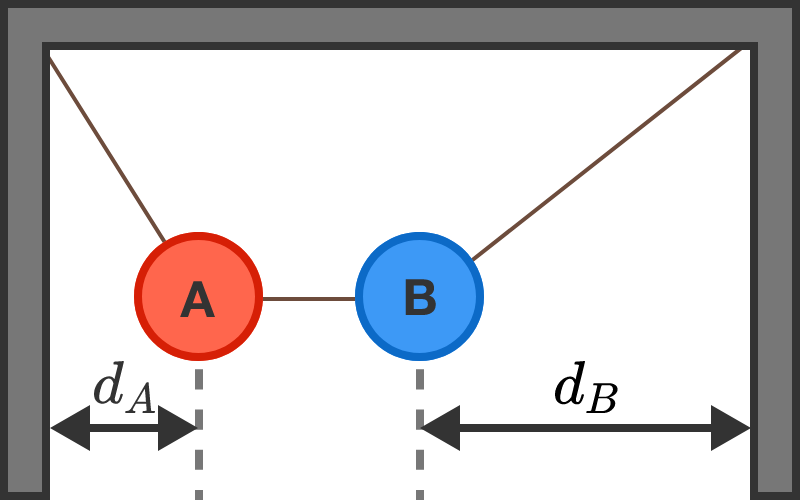# Hanging SpheresAs shown in the diagram, spheres A and B are hanging from the corners of the ceiling, and are connected with a string. The centers of spheres A and B are distances $d_A$ and $d_B$ apart from their respective walls.

What can we say about the respective masses $M_A$ and $M_B$ of the spheres based on the distances $d_A$ and $d_B?$

Assume that the system is in equilibrium, that the string joining the masses is taut and horizontal, that the spheres are the same size, and that $d_B>d_A.$

×